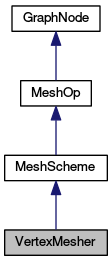MeshKit  1.0
VertexMesher Class Reference

A simple class for meshing geometric vertices. More...

`#include <meshkit/VertexMesher.hpp>`

Inheritance diagram for VertexMesher:[legend]

List of all members.

## Public Member Functions

VertexMesher (MKCore *mkcore, const MEntVector &me_vec=MEntVector())
Bare constructor.
virtual ~VertexMesher ()
Destructor.
Re-implemented here so we can check topological dimension of model_ent.
virtual void setup_this ()
Setup is a no-op, but must be provided since it's pure virtual.
virtual void execute_this ()
The only setup/execute function we need, since meshing vertices is trivial.
virtual const moab::EntityType * mesh_types_arr () const
Return the mesh entity types operated on by this scheme.

## Static Public Member Functions

static const char * name ()
Get class name.
static bool can_mesh (iBase_EntityType dim)
Function returning whether this scheme can mesh entities of t the specified dimension.
static bool can_mesh (ModelEnt *model_ent)
Function returning whether this scheme can mesh the specified entity.
static const moab::EntityType * output_types ()
Get list of mesh entity types that can be generated.

## Private Member Functions

VertexMesher (const VertexMesher &)
No copy constructor, since there's only meant to be one of these.
VertexMesheroperator= (const VertexMesher &)
No operator=, since there's only meant to be one of these.

## Static Private Attributes

static int init
Static variable, used in registration.

## Detailed Description

A simple class for meshing geometric vertices.

INPUT: one or more ModelEnts representing geometric vertices MESH TYPE(S): MBVERTEX OUTPUT: one mesh vertex for each ModelEnt DEPENDENCIES: (none) CONSTRAINTS: ModelEnts must be geometric vertices, i.e. with dimension() == 0

This class performs the trivial task of meshing geometric vertices. Typically there will only be a single instance of this class, and therefore it is pointed to and managed by MKCore. It will also be inserted into the meshing graph during the setup phase of most edge meshers.

The single instance of this class stores all the ModelEnt's representing geometric vertices, and after execution, an MEntSelection entry for each geometric vertex and mesh vertex pair.

Definition at line 33 of file VertexMesher.hpp.

## Constructor & Destructor Documentation

 VertexMesher ( MKCore * mkcore, const MEntVector & me_vec = `MEntVector()` )

Bare constructor.

Definition at line 17 of file VertexMesher.cpp.

 ~VertexMesher ( ) ` [virtual]`

Destructor.

Definition at line 26 of file VertexMesher.cpp.

 VertexMesher ( const VertexMesher & ) ` [private]`

No copy constructor, since there's only meant to be one of these.

## Member Function Documentation

 bool add_modelent ( ModelEnt * model_ent ) ` [virtual]`

Re-implemented here so we can check topological dimension of model_ent.

Parameters:

Reimplemented from MeshOp.

Definition at line 32 of file VertexMesher.cpp.

 static bool can_mesh ( iBase_EntityType dim ) ` [inline, static]`

Function returning whether this scheme can mesh entities of t the specified dimension.

Parameters:
 dim entity dimension

Definition at line 63 of file VertexMesher.hpp.

 static bool can_mesh ( ModelEnt * model_ent ) ` [inline, static]`

Function returning whether this scheme can mesh the specified entity.

Used by MeshOpFactory to find scheme for an entity.

Parameters:
 model_ent ModelEnt being queried
Returns:
If true, this scheme can mesh the specified ModelEnt

Definition at line 72 of file VertexMesher.hpp.

 void execute_this ( ) ` [virtual]`

The only setup/execute function we need, since meshing vertices is trivial.

Implements GraphNode.

Definition at line 45 of file VertexMesher.cpp.

 virtual const moab::EntityType* mesh_types_arr ( ) const` [inline, virtual]`

Return the mesh entity types operated on by this scheme.

Returns:
array terminated with `moab::MBMAXTYPE`

Implements MeshOp.

Definition at line 83 of file VertexMesher.hpp.

 static const char* name ( ) ` [inline, static]`

Get class name.

Definition at line 56 of file VertexMesher.hpp.

 VertexMesher& operator= ( const VertexMesher & ) ` [private]`

No operator=, since there's only meant to be one of these.

 const moab::EntityType * output_types ( ) ` [static]`

Get list of mesh entity types that can be generated.

Returns:
array terminated with `moab::MBMAXTYPE`

Definition at line 14 of file VertexMesher.cpp.

 void setup_this ( ) ` [virtual]`

Setup is a no-op, but must be provided since it's pure virtual.

Implements GraphNode.

Definition at line 42 of file VertexMesher.cpp.

## Member Data Documentation

 int init` [static, private]`

Static variable, used in registration.

Definition at line 97 of file VertexMesher.hpp.

The documentation for this class was generated from the following files: